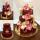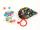# Euler problem

Someone buys a 180 tolars towels. If it was for the same money of 3 more towels, it would be 3 tolars cheaper each. How many were towels?

Result

n =  12

#### Solution:Leave us a comment of example and its solution (i.e. if it is still somewhat unclear...):

Showing 0 comments:Be the first to comment!#### To solve this verbal math problem are needed these knowledge from mathematics:

Looking for help with calculating roots of a quadratic equation? Do you have a system of equations and looking for calculator system of linear equations?

## Next similar examples:

1. SavingsPaul has a by half greater savings than half Stanley, but the same savings as Radek. Staney save 120 CZK less than Radek. What savings have 3 boys together?
2. DollarsMama split 760 dollars Jane, Dane and Eva as follows: Jane got three times more than Dane and Dane and got 40 more than Eva. How much does get each of them?
3. Sweets3 chocolate and 7 cakes cost 85, - CZK. 2 chocolates and 6 cakes cost 86, - CZK. How much is 5 chocolates and 9 cakes? I wonder how to get the result, but only by logic without the use of a system of equations
4. Nine booksNine books are to be bought by a student. Art books cost \$6.00 each and biology books cost \$6.50 each . If the total amount spent was \$56.00, how many of each book was bought?
5. Sheep and cowsThere are only sheep and cows on the farm. Sheep is eight more than cows. The number of cows is half the number of sheep. How many animals live on the farm?
6. LegsCancer has 5 pairs of legs. The insect has 6 legs. 60 animals have a total of 500 legs. How much more are cancers than insects?
7. EquationsSolve following system of equations: 6(x+7)+4(y-5)=12 2(x+y)-3(-2x+4y)=-44
8. Linear systemSolve a set of two equations of two unknowns: 1.5x+1.2y=0.6 0.8x-0.2y=2
9. Equations - simpleSolve system of linear equations: x-2y=6 3x+2y=4
10. BallsMichal said to Martin: give me one ball and I'll have twice as you. Martin said: give me 4 and we will have equally. How many balls each have?
11. Two equationsSolve equations (use adding and subtracting of linear equations): -4x+11y=5 6x-11y=-5
12. Elimination methodSolve system of linear equations by elimination method: 5/2x + 3/5y= 4/15 1/2x + 2/5y= 2/15
13. Three unknownsSolve the system of linear equations with three unknowns: A + B + C = 14 B - A - C = 4 2A - B + C = 0
14. Two numbersWe have two numbers. Their sum is 140. One-fifth of the first number is equal to half the second number. Determine those unknown numbers.
15. Three friendsThe three friends spent 600 KC in a teahouse. Thomas paid twice as much as Paul. Paul a half less than Zdeněk. How many each paid?
16. Linsys2Solve two equations with two unknowns: 400x+120y=147.2 350x+200y=144
17. EquationEquation ? has one root x1 = 8. Determine the coefficient b and the second root x2.